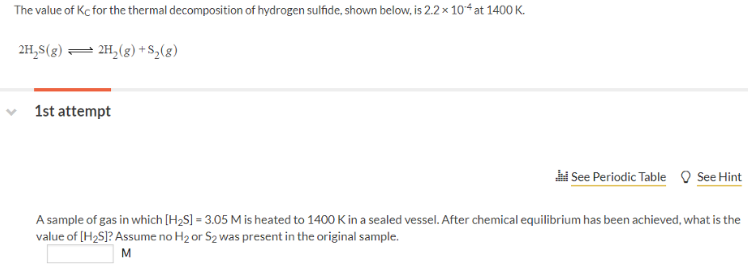# Problem: The value of Kc for the thermal decomposition of hydrogen sulfide, shown below, is 2.2 x 10-4 at 1400 K.2H2S (g) → 2H2 (g) + S2 (g) A sample of gas in which [H2S] = 3.05 M is heated to 1400 K in a sealed vessel. After chemical equilibrium has been achieved, what is the value of [H2S]? Assume no H2 or S2 was present in the original sample.

###### FREE Expert Solution
95% (54 ratings)###### Problem Details

The value of Kc for the thermal decomposition of hydrogen sulfide, shown below, is 2.2 x 10-4 at 1400 K.

2H2S (g) → 2H2 (g) + S2 (g)

A sample of gas in which [H2S] = 3.05 M is heated to 1400 K in a sealed vessel. After chemical equilibrium has been achieved, what is the value of [H2S]? Assume no Hor S2 was present in the original sample.